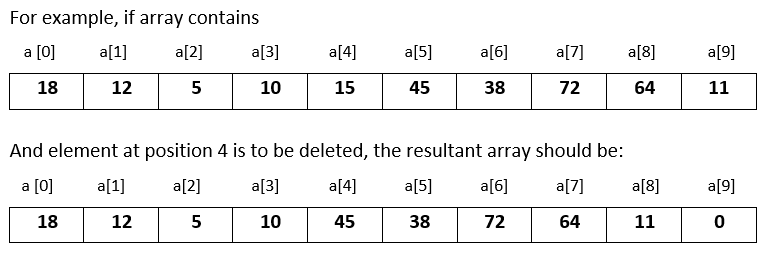# Input 10 numbers in 1d array and delete the number at the given position in C++

###### One Dimensional Array - Question 9

In this question, we will see how to input 10 numbers in a one dimensional integer array and delete a number from the array at a given position in C++ programming. To know more about one dimensional array click on the one dimensional array lesson.

Q9) Write a program in C++ to input 10 numbers in a one dimensional integer array and input a position. Now delete the number at that position by shifting the rest of the numbers to the left and insert a 0 at the end.#### Program

``````#include <iostream>
#include <conio.h>

using namespace std;

int main()
{
int a, i,p;

cout<<"Enter 10 numbers\n";
for(i=0; i<10; i++)
{
cin>>a[i];
}

cout<<"Enter position to delete the number ";
cin>>p;
for(i=p; i<9; i++)
{
a[i]=a[i+1];
}
a[i]=0;

cout<<"\nModified array after deleting the number\n";
for(i=0; i<10; i++)
{
cout<<a[i]<<" ";
}
return 0;
}``````

#### Output

```Enter 10 numbers
18
12
5
10
15
45
38
72
64
11
Enter position to delete the number 4

Modified array after deleting the number
18 12 5 10 45 38 72 64 11 0                ```